TUTORIALS
TUTORIALS HOME

GENERAL MATH
NUMBER SETS
ABSOLUTE VALUE & INEQUALITIES
SETS & INTERVALS
FRACTIONS
POLYNOMIALS
LINEAR EQUATIONS
GEOMETRY
FINITE SERIES
TRIGONOMETRY
EXPONENTS
LOGARITHMS
INDUCTION

CALCULUS
LIMITS
DERIVATIVES
RELATED RATES & OPTIMIZATION
CURVE SKETCHING
INTEGRALS
AREA & VOLUME
INVERSE FUNCTIONS

MAIN
HOME
TESTS
TUTORIALS
SAMPLE PROBLEMS
COMMON MISTAKES
STUDY TIPS
GLOSSARY
CALCULUS APPLICATIONS
MATH HUMOUR

# Derivatives

The average rate of change of a function y=f(x) from x to a is given by the equation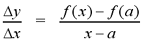The average rate of change is equal to the slope of the secant line that passes through the points (f, f(x)) and (a, f(x)). As x approaches a along the curve, the secant line approaches the tangent line to the curve at a. The slope of the tangent line at a is equal to the instantaneous rate of change of the function at a. This slope can be calculated by taking the limit of the average rate of change, as x approaches a.

A derivative is the instantaneous rate of change of a function. When finding the equation of tangent lines, the slope of the curve f(x) at (a, f(a)) is equal to the derivative of f at a. When finding rates of change, the instantaneous rate of change of a function f(x) when x=a is equal to the derivative of f at a.

Derivatives have their own notation. The derivative of a function f at a number a is denoted by f'(a) and is given by: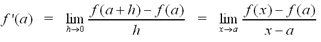So f'(a) represents the slope of the tangent line to the curve at a, or equivalently, the instantaneous rate of change of the function at a.

Note: If we let x=a+h, then as h approaches 0, x will approach a+(0), or simply a. By rearranging x=a+h, we have h=x-a. If we substitute these relations into the limits above, we will find that the two definitions of derivative are equivalent.

# Examples

1 | Find the derivative of the function f
2 | Find the equation of the tangent line to the curve y=f(x) at point P

# Derivative Notations

In addition to the notation above, there are several other notations for expressing the derivative of a function. The most common notations are :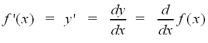The notation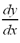is called Leibniz notation. It is the most commonly used notation after the f'(x) notation.

# The Derivative Function

If we find the derivative for the variable x rather than a value a, we obtain a derivative function with respect to x. With this function, the derivative at any value of x can be determined. By replacing a with x in the limit formula, we can find the derivative function.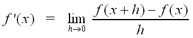# Examples

3 | Find the derivative of the function f at points A, B, C

# Differentiable Functions

A function is differentiable at a if f'(a) exists. It is differentiable on the open interval (a, b) if it is differentiable at every number in the interval.

If a function is differentiable at a then it is also continuous at a. The contrapositive of this theorem states that if a function is discontinuous at a then it is not differentiable at a.

A function is not differentiable at a if its graph illustrates one of the following cases at a :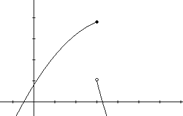Discontinuity

A function is not differentiable at a if there is any type of discontinuity at a. This is stated in the contrapositive of the theorem above. The graph to the right illustrates jump discontinuity.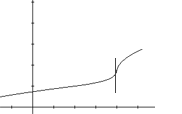Vertical Tangent Line

A function is not differentiable at a if its graph has a vertical tangent line at a. The tangent line to the curve becomes steeper as x approaches a until it becomes a vertical line. Since the slope of a vertical line is undefined, the function is not differentiable in this case.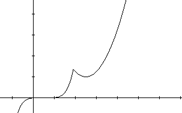Corner

A function is not differentiable at a if its graph has a corner or kink at a. As x approaches the corner from the left- and right-hand sides, the function approaches two distinct tangent lines. Since the function does not approach the same tangent line at the corner from the left- and right-hand sides, the function is not differentiable at that point. The graph to the right illustrates a corner in a graph.

Note: Although a function is not differentiable at a corner, it is still continuous at that point.

# Examples

4 | Find all points where the function is not differentiable
5 | Show that the function is not differentiable at x=a

# Differentiation

Taking limits to find the derivative of a function can be very tedious and complicated. The formulas listed below will make differentiating much easier. Each formula is expressed in the regular notation as well as Leibniz notation.

Constant Rule: If f is a constant function, where f(x) = c, then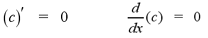Power Rule: If f is a power function, where f(x) = xn and n is a real number, then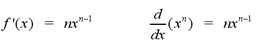Note: The graph of the derivative of a power function will be one degree lower than the graph of the original function.

Note: For an example of a power function question, see Example #6 below.

Constant Multiple Rule: If f is a differentiable function and c is a constant, then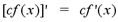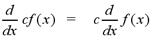Sum Rule: If f and g are differentiable functions, then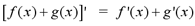In Leibniz notation,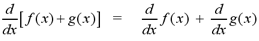Note: For an example of the sum rule, see Example #7 below.

Difference Rule: If f and g are differentiable functions, then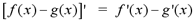In Leibniz notation,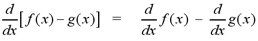Note: For an example of the difference rule, see Example #8 below.

Product Rule: If f and g are differentiable functions, then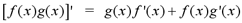In Leibniz notation,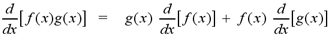Note: For an example of the product rule, see Example #9 below.

Quotient Rule: If f and g are differentiable functions, then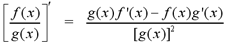In Leibniz notation,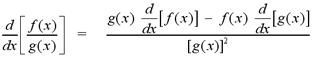Note: For an example of the quotient rule, see Example #10 below.

# Examples

6 | Find the derivative using the power rule
7 | Find the derivative using the sum rule
8 | Find the derivative using the difference rule
9 | Find the derivative using the product rule
10 | Find the derivative using the quotient rule

# The Chain Rule

The chain rule is used to find the derivatives of compositions of functions. A composite function is a function that is composed of two other functions. For the two functions f and g, the composite function or the composition of f and g, is defined by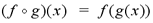The function g(x) is substituted for x into the function f(x). For example, the function F(x)=(2x+6)4 could be considered as a composition of the functions, f(x)=x4 and g(x)=2x+6. However, it could also be written as a composition of f(x)=(2x)4 and g(x)=x+3. Often, a function can be written as a composition of several different combinations of functions.

The chain rule allows us to find the derivative of composite functions. The chain rule states that if f and g are differentiable functions and F(x)=f(g(x)), then F is differentiable and the derivative of F is given by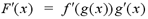In Leibniz notation, if y=f(u), u=g(x) and y and u are differentiable functions, then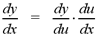# Examples

11 | Find the derivative using the chain rule

# Derivatives of Trigonometric Functions

The limits below are required for proving the derivatives of trigonometric functions. These limits and the derivatives of the trigonometric functions will be proven in your calculus lectures. Here, they are simply stated.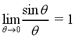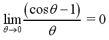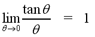Note: These limits are used often when solving trigonometric limit problems. Try to remember them and the conditions under which they hold.

The derivatives of the six trigonometric functions are shown below.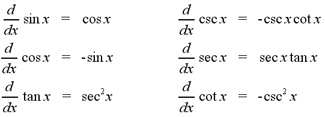Note: The derivatives of the co-functions (cosine, cosecant and cotangent) have a "-" sign at the beginning. The derivatives of the other 3 functions have a "+" sign at the beginning. This is a helpful way to remember the signs when taking the derivatives of trigonometric functions.

# Examples

12 | Find the derivative of the trigonometric function
13 | Evaluate the limit

# Implicit Differentiation

The method of implicit differentiation allows us to find the derivative of an implicit function. It allows us to differentiate y without solving the equation explicitly. We can simply differentiate both sides of the equation and then solve for y'. When differentiating a term with y, remember that y is a function of x. The term is a composition of functions, so we use the chain rule to differentiate. For example, if you were to differentiate the term 3y4 it would become (12y3)(y').

Note: For a more concrete demonstration of how to differentiate implicit functions, see example #14 below.

# Examples

14 | Find the derivative by implicit differentiation

# Higher Derivatives

Earlier in the derivatives tutorial, we saw that the derivative of a differentiable function is a function itself. If the derivative f' is differentiable, we can take the derivative of it as well. The new function, f'' is called the second derivative of f. If we continue to take the derivative of a function, we can find several higher derivatives. In general, f (n) is called the nth derivative of f.

Note: Recall that when working with motion application problems, the velocity of the particle is the first derivative of the displacement function. The acceleration of the particle is the derivative of the velocity function, or equivalently, the second derivative of the displacement function.

# Examples

15 | Find the higher derivatives of y
16 | Find the first and second derivatives of the function

# Linear Approximation

It is often easy to calculate the exact value of a function at a point a, but rather difficult to compute values near a. For example, Ö9 is quite easy to calulate while Ö8.94 is rather difficult to calculate.

We can find an approximate value of the function at points near a by using the tangent line to the curve at a. If the function y=f(x) is differentiable at a, then the linear approximation (or linearization) of f at a is given by :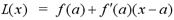# Examples

17 | Find the linear approximation of f at a

For more practice with the concepts covered in the derivatives tutorial, visit the Derivatives Problems page at the link below. The solutions to the problems will be posted after the derivatives chapter is covered in your calculus course.

To test your knowledge of derivatives, try taking the general derivative test on the iLrn website or the advanced derivative test at the link below.

Derivative Problems
General Derivative Test on iLrn

| Top of Page |

COURSE HOMEPAGES
MATH 1036
MATH 1037

FACULTY HOMEPAGES
Alex Karassev
Ted Chase